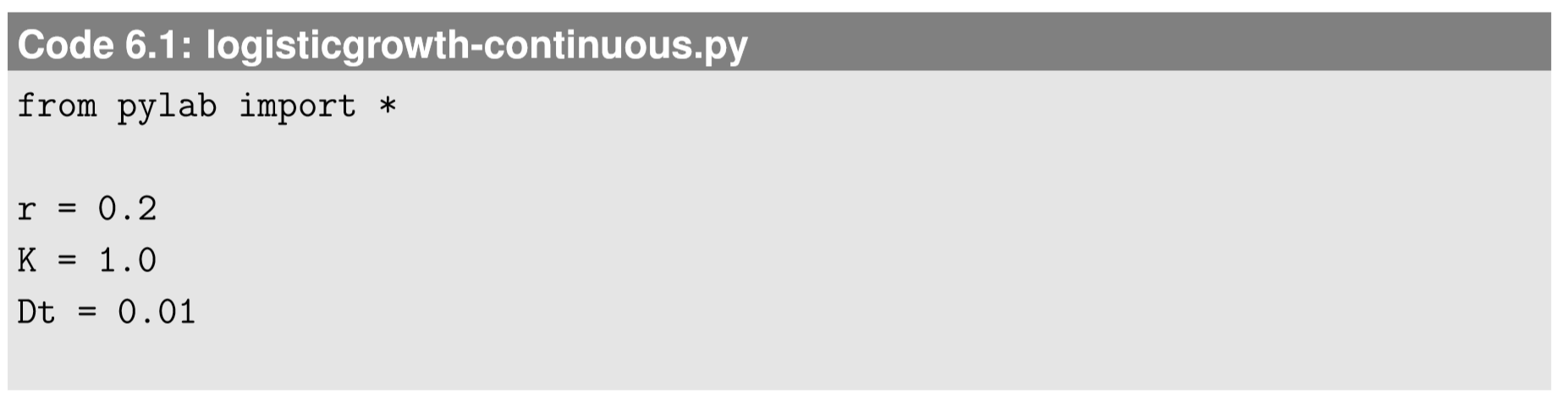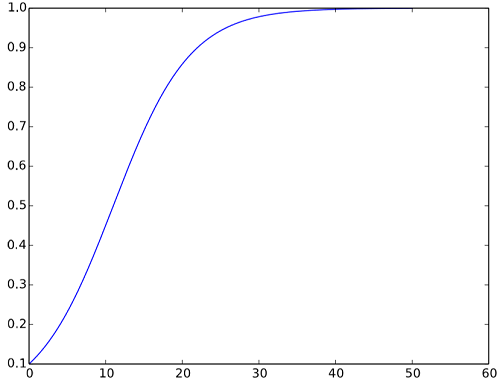$$\newcommand{\id}{\mathrm{id}}$$ $$\newcommand{\Span}{\mathrm{span}}$$ $$\newcommand{\kernel}{\mathrm{null}\,}$$ $$\newcommand{\range}{\mathrm{range}\,}$$ $$\newcommand{\RealPart}{\mathrm{Re}}$$ $$\newcommand{\ImaginaryPart}{\mathrm{Im}}$$ $$\newcommand{\Argument}{\mathrm{Arg}}$$ $$\newcommand{\norm}{\| #1 \|}$$ $$\newcommand{\inner}{\langle #1, #2 \rangle}$$ $$\newcommand{\Span}{\mathrm{span}}$$

6.4: Simulating Continuous-Time Models

$$\newcommand{\vecs}{\overset { \rightharpoonup} {\mathbf{#1}} }$$

$$\newcommand{\vecd}{\overset{-\!-\!\rightharpoonup}{\vphantom{a}\smash {#1}}}$$

Simulation of a continuous-time model is equivalent to the numerical integration of differential equations, which, by itself, is a major research area in applied mathematics and computational science with more than a century of history. There are a large number of methodologies developed for how to accomplish accurate, fast, efﬁcient numerical integrations. It would easily take a few books and semesters to cover them, and this textbook is not intended to do that. Instead, here we focus on the simplest possible method for simulating a continuous time model, by using the following formula as an approximation of a differential equation

\begin{align} \dfrac{dx}{dt} &=G(x); \\[4pt] x(t+\Delta{t}) &= x(t) +G(x(t))\Delta{t} \label{6.26} \end{align}

This method is called the Euler forward method. Its basic idea is very straightforward; you just keep accumulating small increases/decreases of $$x$$ that is expected from the local derivatives speciﬁed in the original differential equation. The sequence produced by this discretized formula will approach the true solution of the original differential equation at the $$\displaystyle \lim_{∆t → 0}$$, although in practice, this method is less accurate for ﬁnite-sized $$∆t$$ than other more sophisticated methods (see Exercise 6.6.3). Having said that, its intuitiveness and easiness of implementation have a merit, especially for those who are new to computer simulation. So let’s stick to this method for now.

In nearly all aspects, simulation of continuous-time models using the Euler forward method is identical to the simulation of discrete-time models we discussed in Chapter 4. Probably the only technical difference is that we have $$∆t$$ as a step size for time, which may not be 1, so we also need to keep track of the progress of time in addition to the progress of the state variables. Let’s work on the following example to see how to implement the Euler forward method.

Here we consider simulating the following continuous-time logistic growth model for $$0 ≤ t < 50$$ in Python, with $$x(0) = 0.1$$, $$r = 0.2$$, $$K = 1$$ and $$∆t = 0.01$$:

$\frac{dx}{dt} =rx(1-\frac{x}{K}) \label{6.27}$

The ﬁrst (and only) thing we need to do is to discretize time in the model above. Using Eq. \ref{6.26}, the equation becomes

$x(t+\Delta{t}) =x(t) +rx(t)(1 -\frac{x(t)}{K}) \Delta{t}, \label{6.28}$

which is nothing more than a typical difference equation. So, we can easily revise Code 4.10 to create a simulator of Eq. \ref{6.27}:Note that there really isn’t much difference between this and what we did previously. This code will produce a nice, smooth curve as a result of the numerical integration, shown in Figure 6.4.1. If you choose even smaller values for $$∆t$$, the curve will get closer to the true solution of Eq. \ref{6.27}.Figure $$\PageIndex{1}$$: Visual output of Code 6.1.

Exercise $$\PageIndex{1}$$

Vary $$∆t$$ to have larger values in the previous example and see how the simulation result is affected by such changes.

As the exercise above illustrates, numerical integration of differential equations involves some technical issues, such as the stability and convergence of solutions and the possibility of “artifacts” arising from discretization of time. You should always be attentive to these issues and be careful when implementing simulation codes for continuous-time models. I hope the following exercises will help you further investigate those subtleties of the numerical integration of differential equations.

Exercise $$\PageIndex{2}$$: Lotka-Volterra (predator prey) model

Simulate the following continuous-time Lotka-Volterra (predator prey) model for $$0 ≤ t < 50$$ in Python, with $$x(0) = y(0) = 0.1$$, $$a = b = c = d = 1$$ and $$∆t = 0.01$$. Visualize the simulation results over time and also in a phase space.

$\frac{dx}{dt} =ax-bxy \label{6.29}$

Exercise $$\PageIndex{3}$$: Other integration methods

There are many other more sophisticated methods for the numerical integration of differential equations, such as the backward Euler method, Heun’s method, the Runge-Kutta methods,etc. Investigate some of those methods to see how they work and why their results are better than that of the Euler forward method.